## Facebook job puzzles: Prime bits

by Jesse Farmer on Tuesday, April 15, 2008

Welcome to the second installment of 20bits' Facebook job puzzles solution manual. This time I am going to tackle the Prime Bits puzzle.

Like my Korn Shell solution, this puzzle is mostly mathematical. This time, however, we're going to be wading deep into combinatorics territory. Combinatorics is the mathematics of counting. If you have three pairs of socks, two pairs of pants, and four shirts, how many outfits can you wear? If you have a collection of twenty playing cards how many two-card hands are there? These are the sorts of questions combinatorics exists to answer, although the questions quickly become more complicated.

Again, I'm aiming to make this understandable to an intelligent layperson interested in the puzzle. If that's you then read on!

#### The Question

This time around the question is much more straightforward. Every (positive) integer can be represented using binary. Normally we write using decimal, which is based around powers of ten. For example, 215 is really 2*102 + 1*101 + 5*100. Binary involves using 2s rather than 10s as place values, so, e.g., 5 is written as 101 = 1*22 + 0*21 + 1*20.

Every integer therefore can be respresented as a string of zeroes and ones. Define P(x) to be true if the number of ones in the binary representation of x is prime and false otherwise. So, e.g., P(5) is true but P(4) is false. Our job is to implement the function uint64_t prime_bits(uint64_t a, uint64_t b); which returns the number of integers k, a ≤ k ≤ b such that P(k) is true. uint64_t is a way of designating 64-bit numbers in C/C++.

#### The Obvious Solution

Assuming we have implemented P(x) the obvious solution in Python is this

```def prime_bits(a,b):
return sum([P(k) for k in range(a,b+1)]);```

That is, for each integer in the desired range we calculate P(k) and them sum all these values. Since "true" corresponds to "1" and "false" to "0" we get the total number of true entries in our desired range. A more explicit procedural implementation would be

```def prime_bits(a,b):
c = 0
for k in range (a,b+1):
if P(k):
c = c + 1
return c```

Assuming P(n) = O(n) (this is called Big-O notation) then we have prime_bits(a,b) = O(b2). Surely we can do better. In fact, according to the constraints on the Facebook page we have to do better to even be considered in the running.

The above big-o analysis tells us that we must be careful of two things: one, our imlementation, whatever it is, cannot iterate over every integer between a and b; two, our prime-checking function has to be fast enough that it doesn't dwarf the running time of the rest of our algorithm.

#### Bring a Little Binomial into Your Life

Forget about the fact that we're dealing with numbers and think of our binary representation as a string. That is, think of "110101" as nothing more than some sequence of zeroes and ones. It could just as easily be "aababa" or "!!?!?!" or any other two characters we choose.

From this perspective we can turn the question on its head. Rather than going through each possible string one by one, let's count groups of strings en masse. How many 6-character strings of zeroes and ones have 3 ones?

The answer rests in that foundational function of combinatorics, the binomial coefficient. It is defined as follows: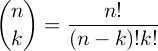pronounced "n choose k" and sometimes written nCk, where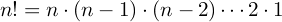is the factorial.

This might seem like gibberish so let's start with what it means. Let's say we're given a vat of balls labeled from 1-6. The binomial coefficient tells us how many ways we can pick three balls from the vat if we don't care about the order in which they are picked. We might choose {1,2,3} or {7,3,9}, for example, but {4,5,3} and {3,4,5} are considered to be the same drawing.

How might we go about counting this? Well, let's start by caring about order because that's easier to count. If we do care about order then there are 6 ways to pick the first ball, 5 ways to pick the second ball, and 4 ways to pick the third and final ball. This means there are 6*5*4 ways to pick the balls if we care about order, i.e., if we treat (4,5,3) and (3,4,5) as different drawings.

Now how many ways are there to arrange each triplet? Well, there are 3 ways to choose the first element, 2 ways to choose the second, and 1 way to choose the third and final element. Explicitly, the six following triplets are different orderings of the same drawing:

```(1,2,3), (2,3,1), (3,1,2), (1,3,2), (3,2,1), (2,1,3)
```

But we can write 6*5*4 = 6!/3! and 3*2*1 = 3!, using the factorial notation. This means that if we don't care about order, there are a total of 6!/(3!*3!) ways of drawing balls from the vat. Or we could write (6!/(6-3)!3!) = 6C3, 6 choose 3. So hopefully you can see that the above formula didn't totally come from nowhere.

Let's go back to our binary brou-ha-ha. Let's take a 6-bit binary string, 000000. Now, how many such strings have three ones? Well, 6C3. If we want to know how many 6-bit binary strings have a prime number of ones we just need to find all the prime numbers less than 6 and use the binomial coefficient. Since 2, 3, and 5 are the only primes less than 6 we know there are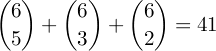6-bit binary strings with either 2, 3 or 5 (i.e., a prime number of) ones.

#### Paging Mr. Pascal

Since our motivation for writing a new method is performance the above might very well be worthless if we can't make it perform. Luckily the binomial coefficient has an excellent recursive definition related to Pascal's triangle: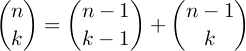By using dynamic programming we can generate the nth row of Pascal's triangle in O(n2) time. But wait, weren't we looking for something that beat this? (Remember our original implementation of prime_bits(a,b) took O(b2) time.) Here's where you need to be careful: we need to generate as many rows in Pascal's triangle as there are significant bits in our integer. For a 64-bit integer this means we'll be generating at most 64 rows of Pascal's triangle. In terms of our inputs a and b this is O((log b)2) time. Of course we're a far cry from a full implementation, but at least we know we can use binomial coefficients within our performance requirements.

#### Back to Numbers

Let's say we have a number which is a power of two, e.g., 28. In binary this is 10000000. All numbers less than it are of the form 0xxxxxxxx, where x is either 0 or 1. We have eight places to fill and we're interested in those combinations which have a prime number of 1s. 2, 3, 5, and 7 are the only primes less than 8 so there are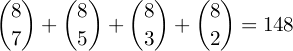positive integers less than 28 which have a prime number of ones in their binary representation.

So far so good, but the above method only works for numbers that are powers of two. What happens if we want to count the number of desired positive integers less than 100101? 111101 has a prime number of ones but it is larger than 100101. So how do we extend the above method to arbitrary integers?

Consider the following three ranges of numbers:

```Numbers less than 100101 (37)
----
000000 to 011111
100000 to 100011
100100
100101
```

First, does this range of numbers encompass every number less than 100101? The first range is the set of all numbers less than 100000, the second the set of all numbers less than 100100, the third the set of all numbers less than 100101, and the fourth is the number itself. So certainly every number in this set is less than 100101, but is that every such number?

Yes, and we can see that by looking at place values. If a number agrees with 100101 in the lest-most bit then it cannot have a 1 in the next two places without being greater than the number in question. Likewise, if it agrees with 100101 in the for the four left-most bits then it cannot have a 1 in the second position without being greater.

From this we can determine combinatorially how many such numbers have a prime number of 1 bits. For the range 000000 to 011111 there are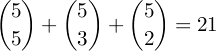numbers which have the desired property since we have 5 bits to choose freely and 2,3, and 5 are the only primes less than or equal to 5.

The next range, 100000 to 10011, is similar, except we have one bit fixed and two bits to choose freely. Thus there are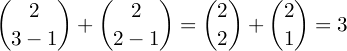numbers which have the desired property. This is because we there is already one bit set, so we count all combinations which, when added to 1, have a prime number of bits set to 1.

#### The Algorithm and Some Caveats

So, for a given number we now have a function pb(n) which counts the number of positive integers less than or equal to n which have a prime number of bits set to 1. prime_bits(a,b) then just becomes pb(b) - pb(a).

To find the number of positive integers between 1 and n, inclusive, we therefore need three things:

1. Generate Pascal's triangle so we can easily extract binomial coefficients. This takesO((log n)2) time.
2. Given a number n find its most significant (or left-most) bit. This takes O(log n) time.
3. For each bit set to 1 count the number of combinations of bits to its right which yield a prime number of bits. If we have a pre-generated list of primes this takes O((log n)2), otherwise we can do it in O((log n)3) time.

Although the Facebook puzzle says it would be "uncouth" to not support integers over 64 bits, generating lists of primes is a well-understood problem. Using the Sieve of Eratosthenes one can generate every prime less than k in O(k) time and using the Sieve of Atkin one can do it in O(k/log log k) time. For now I'm just going to hard-code every prime less than 1024. This means the above algorithm will work for up to 1024-bit integers. If you care about running the above algorithm for abritrarily large numbers, well, you can implement one of the prime number sieves yourself.

Here is my implementation in Python.

This code has been redacted at the request of Facebook. Contact me if you want the code.

#### Improvements

The code as-is runs quite fast. Every input I've given it runs in well under a second, usually less than a tenth of a second, but there are still improvements.

1. Rather than calculate pb(b) - pb(a), integrate the two passes by determining the first bit-position in which a and b differ and running a variation of the above counting algorithm.
2. Improve the significant bit calculation. The current implementation is the most naÃ¯ve method.
3. Generally tweak the loops to improve speed, since Python is notoriously slow in iterating over lists. Or write it in a langage like C (or ASM, you hardcode d00d, you).
4. Anyhow, none of the above strike me as particularly "interesting," so I'll leave them to more enterprising individuals. Cheers and happy coding!